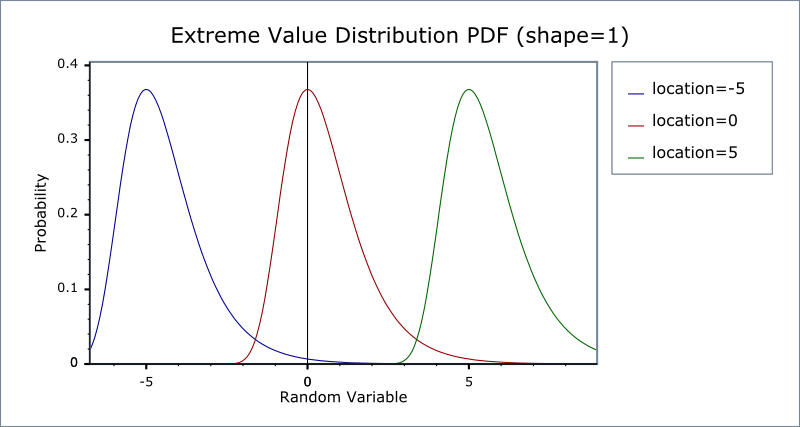#Boost C++ Libraries

...one of the most highly regarded and expertly designed C++ library projects in the world.

This is the documentation for an old version of boost. Click here for the latest Boost documentation.
##### Extreme Value Distribution

`#include <boost/math/distributions/extreme.hpp>`

```template <class RealType = double,
class Policy   = policies::policy<> >
class extreme_value_distribution;

typedef extreme_value_distribution<> extreme_value;

template <class RealType, class Policy>
class extreme_value_distribution
{
public:
typedef RealType value_type;

extreme_value_distribution(RealType location = 0, RealType scale = 1);

RealType scale()const;
RealType location()const;
};
```

There are various extreme value distributions : this implementation represents the maximum case, and is variously known as a Fisher-Tippett distribution, a log-Weibull distribution or a Gumbel distribution.

Extreme value theory is important for assessing risk for highly unusual events, such as 100-year floods.

More information can be found on the NIST, Wikipedia, Mathworld, and Extreme value theory websites.

The relationship of the types of extreme value distributions, of which this is but one, is discussed by Extreme Value Distributions, Theory and Applications Samuel Kotz & Saralees Nadarajah.

The distribution has a PDF given by:

f(x) = (1/scale) e-(x-location)/scale e-e-(x-location)/scale

Which in the standard case (scale = 1, location = 0) reduces to:

f(x) = e-xe-e-x

The following graph illustrates how the PDF varies with the location parameter:And this graph illustrates how the PDF varies with the shape parameter:##### Member Functions
```extreme_value_distribution(RealType location = 0, RealType scale = 1);
```

Constructs an Extreme Value distribution with the specified location and scale parameters.

Requires ```scale > 0```, otherwise calls domain_error.

```RealType location()const;
```

Returns the location parameter of the distribution.

```RealType scale()const;
```

Returns the scale parameter of the distribution.

##### Non-member Accessors

All the usual non-member accessor functions that are generic to all distributions are supported: Cumulative Distribution Function, Probability Density Function, Quantile, Hazard Function, Cumulative Hazard Function, mean, median, mode, variance, standard deviation, skewness, kurtosis, kurtosis_excess, range and support.

The domain of the random parameter is [-∞, +∞].

##### Accuracy

The extreme value distribution is implemented in terms of the standard library `exp` and `log` functions and as such should have very low error rates.

##### Implementation

In the following table: a is the location parameter, b is the scale parameter, x is the random variate, p is the probability and q = 1-p.

Function

Implementation Notes

pdf

Using the relation: pdf = exp((a-x)/b) * exp(-exp((a-x)/b)) / b

cdf

Using the relation: p = exp(-exp((a-x)/b))

cdf complement

Using the relation: q = -expm1(-exp((a-x)/b))

quantile

Using the relation: a - log(-log(p)) * b

quantile from the complement

Using the relation: a - log(-log1p(-q)) * b

mean

standard deviation

pi * b / sqrt(6)

mode

The same as the location parameter a.

skewness

12 * sqrt(6) * zeta(3) / pi3

kurtosis

27 / 5

kurtosis excess

kurtosis - 3 or 12 / 5